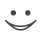This flashcard is just one of a free flashcard set. See all flashcards!

49
The zero curve is upward sloping. Define X as the 1-year par yield, Y as the 1-year zero rate and Z as the forward rate for the period between 1 and 1.5 year. Which of the following is true?
A.X is less than Y which is less than Z
B.Y is less than X which is less than Z
C.X is less than Z which is less than Y
D.Z is less than Y which is less than X
E.X is less than Y which is less than Z
F.Y is less than X which is less than Z
G.X is less than Z which is less than Y
H.Z is less than Y which is less than X